# How to increase resolution in ANY-OUT programmable output voltage devices

With an adjustable LDO, you could create any output voltage. However, this is not the case with ANY-OUT programmable output voltage devices such as the TPS7A8300, the TPS7A7100, TPS7A7200 and TPS7A7300, where both range and resolution are limited.  With these four devices, you can use their common-feedback architecture to improve resolution.

Common-feedback architecture highlighted in the figure below: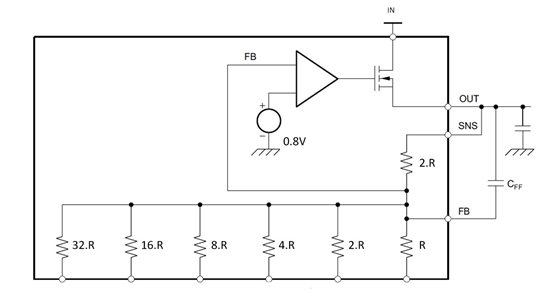Figure 1: ANY-OUT architecture

I am using the values shown for TPS7A83 as an example.  Notice that the reference voltage is, essentially, amplified by the gain around the amplifier.  The feedback resistor here is set to 2.R and the gain resistors can be selected.  Furthermore, the gain resistors are binary weighted.  If the gain resistor of value R is connected to GND, then the gain seen by the reference voltage will be 1+(2.R)/R=3 and the output will regulate to 0.8V ×3= 2.4V.

Since the resistors are binary weighted, each gain resistor, if connected to GND, individually will have a contribution of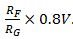.  Using superposition, it then becomes possible to determine the output voltage when multiple resistances are connected to ground.

The minimum resolution is given by the largest gain resistor.  In the case of the TPS7A83, the minimum resolution is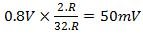.

Note that so far, there is nothing new as all this information is directly available from the datasheet.  Looking at table 2 of the TPS7A83 datasheet will give you the output voltage corresponding to the combination of resistors connected to ground.

Let’s go a little further and see how to expand on this.

The four devices mentioned at the beginning also have the particularity of bringing both the sense and the feedback pin outside.  Let’s first look at how to use the sense pin to increase the output voltage resolution.

There are two settings of potential interest to increase the output voltage resolution, the first one will help achieve the finest resolution possible using on-chip component, the other increases the resolution by adding one external resistor.

Figure 2 shows the first circuit configuration.  By connecting in parallel the 2.R  feedback resistor in parallel with the smallest gain resistor, a new feedback resistor with a value of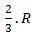is created.  This resistor value combined with the largest gain resistor of 32.R  will provide a 16.67mV resolution for the TPS7A83xx.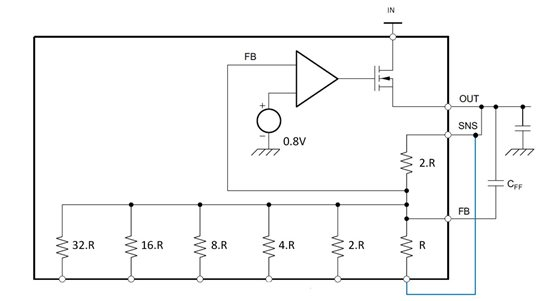Figure 2: Increased resolution circuit #1

The output voltage range of this configuration has been reduced from the original [0.8V +3.95V] to [0.8V +1.317V].  Note that the ±1% accuracy of the TPS7A8300 as set by the internal resistor network translates to a minimum voltage accuracy of ±8mV.  This is still at least two times better than the ANY-OUT programmable voltage resolution.

This configuration has another variant when paralleling the 2.R feedback resistance with the 2.R gain resistance to achieve a minimum resolution of 25mV is the programmable voltage.  However, it has the inconvenience of splitting the range accessible by ANY-OUT programmation into two.  For the 25mV resolution, the range is [0.8 1.175V] and [1.6V 1.975V].

The 2nd circuit configuration takes advantage of the fact that the feedback pin is available, allowing you to connect additional gain setting resistance.  By adding a 64.R resistor, the resolution in increased to 25mV.  A 128.R resistor will increase the resolution to 12.5mV, which is what I would consider to be the limit of programmability due to the device accuracy for the TPS7A8300.

Now, look at the resistor values, as well as thermal gradient matching between the external and internal resistor, to simultaneously achieve both the original voltage range and the increased resolution.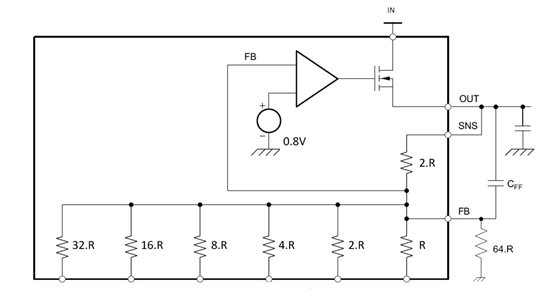Figure 3: Increased resolution circuit #2

With these two different approaches, you can increase the programmability resolution and minimize the number of required external components. If you have any questions, please leave them in the comment section below.

• Hello,

Yes, resistor tolerance are not listed since the ANYOUT is intended to be used without adding any external resistor and excellent resistor matching is achieved.

As mentioned previously, the resistance tolerance on semiconductor process is poor, however, when the same size resistor is used many time on the same silicon, the geometry of each resistor in the silicon will be the same, as each resistor are patterned at the same time.  In that fashion, all the resistors may be off if taken individually by a large absolute error, but will have an excellent resistor to resistor matching.  Hence the ratio between the resistor will stay very accurate from chip to chip.

Regards,

Xavier

• HI there

Thx. For the third case, I don't see the 15% tolerances for internal resistors anywhere in the datasheet. The datasheet states that 1% voltage accuracy (electrical characteristics). It also recommends using internal resistors as they are matched to 1% (page 19), Could you please clarify